Next: The Hartree-Fock-Bogolyubov equations Up: The Skyrme Hartree-Fock-Bogolyubov equations Previous: The Hartree-Fock-Bogolyubov energy

## The Hartree-Fock-Bogolyubov mean fields

Since the Bogolyubov transformation does not preserve the particle number, we introduce two Lagrange multipliers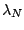and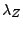to conserve the average neutron and proton number. The HFB equations are then obtained by writing the stationary condition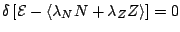. The dependence of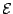on the kinetic densities leads the effective mass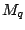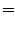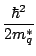(22)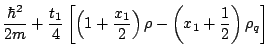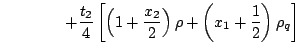(23)

and to the abnormal effective mass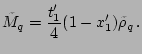(24)

The particle-hole (Hartree-Fock) fields is given by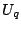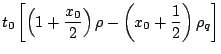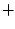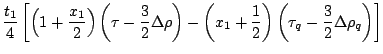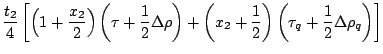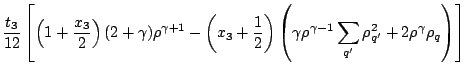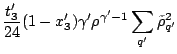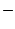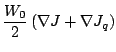(25)

In the case of protons, varying the expressions (21) and (22) leads to the following expression for the Coulomb field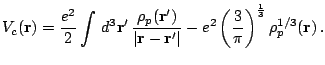(26)

The particle-particle (pairing) field is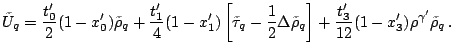(27)

Finally, the spin-orbit fields (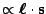) have the following form factors: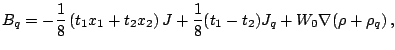(28)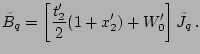(29)Next: The Hartree-Fock-Bogolyubov equations Up: The Skyrme Hartree-Fock-Bogolyubov equations Previous: The Hartree-Fock-Bogolyubov energy
Jacek Dobaczewski 2005-01-23